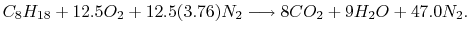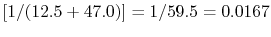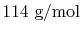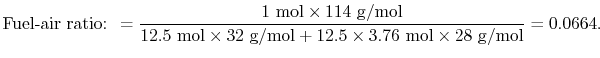Thermodynamics and Propulsion

15.2 Fuel-Air Ratio

The reaction for aeroengine fuel at stoichiometric conditions isOn a molar basis, the ratio of fuel to air is.

To find the ratio on a mass flow basis, which is the way in which the aeroengine industry discusses it, we need to weight'' the molar proportions by the molecular weight of the components. The fuel molecular weight is, the oxygen molecular weight isand the nitrogen molecular weight is (approximately). The fuel/air ratio on a mass flow basis is thusIf we used the actual constituents of air we would get 0.0667, a value about 0.5% different.

Muddy Points

Do we always assume 100% complete combustion? How good an approximation is this? (MP 15.3)

UnifiedTP